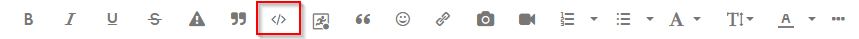## Create a quoted list separated by commas

Hi All, below is the code to create a quoted list separated by spaces. I am trying to modify the program using translate function to create a new list in the same code which contains the output is a list separated by commas. But, what I am trying is not working.

Please, help me how to correct this error.

Here, is the code that creates a quoted list separated by spaces:

data one;
input empl \$;
datalines;
12345
67890
45678
;

%let list=;
data _null_;
set one;
call symputx('mac',quote(strip(empl)));
call execute('%let list=&list &mac');
run;

%put &=list;

Output in the log:

%put &=list;
LIST="12345" "67890" "45678"

Now, I am trying to use this LIST in the same program to create a new list separated by commas . Here, is the code that I am trying:

%let list=;
%let var1=;
data _null_;
set one;
call symputx('mac',quote(strip(empl)));
call execute('%let list=&list &mac');
call execute('%let var1=\$sysfunc(translate(&list,',',' '))');
run;

%put &=list;
%put &=var1;

In the log errors are:

59 call execute('%let var1=\$sysfunc(translate(&list,',',' '))');
----
22
-------
253
ERROR 22-322: Syntax error, expecting one of the following: !, !!, &, *, **, +, ',', -, /, <,
<=, <>, =, >, ><, >=, AND, EQ, GE, GT, LE, LT, MAX, MIN, NE, NG, NL, OR, ^=, |,
||, ~=.

ERROR 253-185: The EXECUTE subroutine call has too many arguments.

60 run;

NOTE: The SAS System stopped processing this step because of errors.
NOTE: DATA statement used (Total process time):
real time 0.01 seconds
cpu time 0.00 seconds

61
62 %put &=list;
LIST=
63 %put &=var1;
VAR1=

1 ACCEPTED SOLUTION

Accepted Solutions

## Re: Create a quoted list separated by commas

You can skip the CALL SYMPUTX() function in your first code since you don't seem to need that second macro variable.

``````%let list=;
data _null_;
set one;
call execute(catx(' ','%let list=&list', quote(trim(empl)),';'));
run;

%put &=list;``````

If you want to add commas you need to treat the first one differently than the second one to avoid adding a comma at the front.

``````data _null_;
set one;
if _n_=1 then call symputx('list',quote(trim(empl)));
else call execute(catx(',','%let list=&list', quote(trim(empl)) )||';');
run;

%put &=list;``````

Why do you want to add the commas?  The IN operator in SAS code does not need them.

To SAS these two statements are the same:

``````where empl in ('1234' '3456');
where empl in ('1234','3456');
``````
7 REPLIES 7

## Re: Create a quoted list separated by commas

A macro function (like SYSFUNC) needs to be called with %, not with \$.

But you can achieve your requested result much easier:

``````proc sql noprint;
select quote(strip(empl)) into :mac separated by ","
from one;
quit;``````

## Re: Create a quoted list separated by commas

Thank you very much. It worked for me.

## Re: Create a quoted list separated by commas

We need the ENTIRE log, not just the errors. This has been mentioned to you before.

Also, we need this log pasted into the window that appears when you click on the </> icon.--
Paige Miller

## Re: Create a quoted list separated by commas

You can skip the CALL SYMPUTX() function in your first code since you don't seem to need that second macro variable.

``````%let list=;
data _null_;
set one;
call execute(catx(' ','%let list=&list', quote(trim(empl)),';'));
run;

%put &=list;``````

If you want to add commas you need to treat the first one differently than the second one to avoid adding a comma at the front.

``````data _null_;
set one;
if _n_=1 then call symputx('list',quote(trim(empl)));
else call execute(catx(',','%let list=&list', quote(trim(empl)) )||';');
run;

%put &=list;``````

Why do you want to add the commas?  The IN operator in SAS code does not need them.

To SAS these two statements are the same:

``````where empl in ('1234' '3456');
where empl in ('1234','3456');
``````

## Re: Create a quoted list separated by commas

Thank you very much. Both the solutions worked for me. Now, I understand how to achieve this in the data step.

I didn't understand why ';' and ||';' in catx, but without using these also, it worked. Can you please help me understand why to use ';' and ||';' in catx.

As you said though both with or without commas are treated as same, but I want to learn how to do this.

## Re: Create a quoted list separated by commas

@Moksha wrote:

Thank you very much. Both the solutions worked for me. Now, I understand how to achieve this in the data step.

I didn't understand why ';' and ||';' in catx, but without using these also, it worked. Can you please help me understand why to use ';' and ||';' in catx.

As you said though both with or without commas are treated as same, but I want to learn how to do this.

Must be some "feature" of CALL EXECUTE() when you only send it macro code.  Notice how you should have a NOTE in the log that call execute worked but no SAS code was generated.  I wouldn't count on it working that way in the future as it seems like a BUG to me.

## Re: Create a quoted list separated by commas

Probably, a bug. Yes, there is a NOTE in the log that call execute worked but no SAS code was generated.

Discussion stats
• 7 replies
• 764 views
• 3 likes
• 4 in conversation